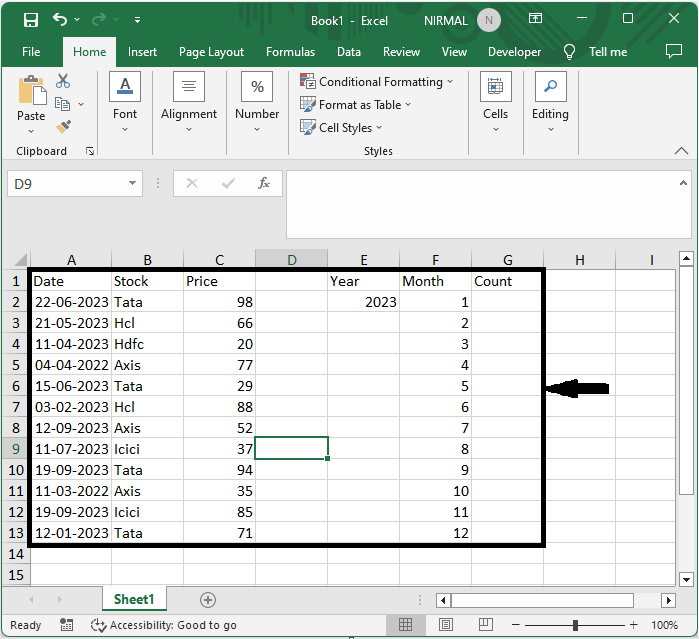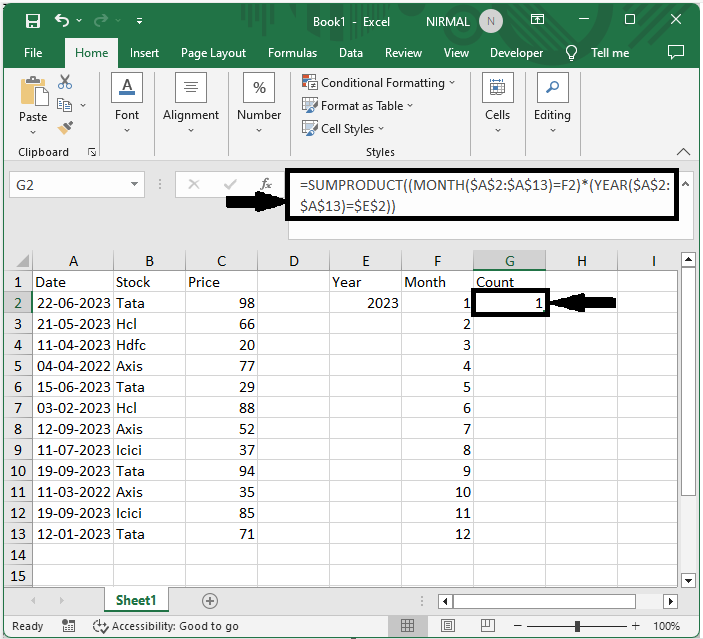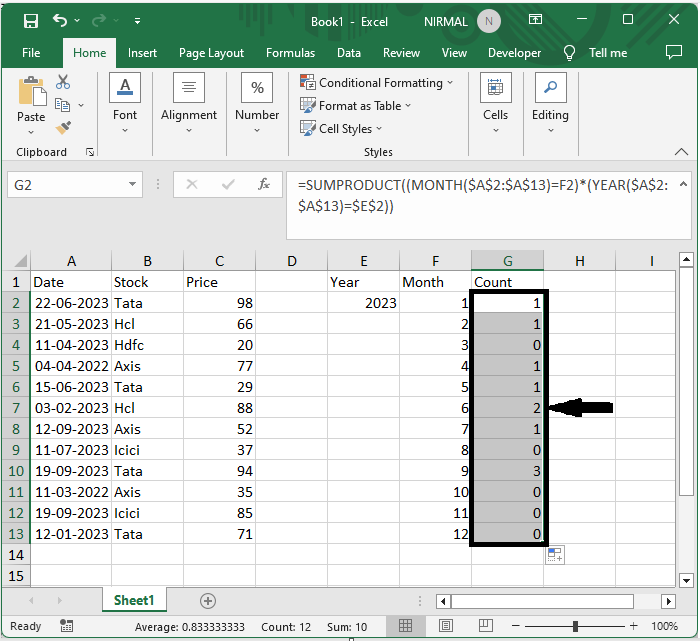# How to Count the Number of Occurrences Per Year/Quarter/Month/Week in Excel?

In many industries, including finance, sales, and project management, tracking and evaluating data based on time intervals is a typical activity. Excel offers a productive way to handle this difficulty with its robust features and functionalities.

This article will take you step-by-step through the process of setting up your data, using the proper formulas, and producing precise count results, regardless of your level of Excel proficiency. By the end of this tutorial, you will have a firm grasp on how to use Excel's features to effectively count occurrences throughout various time periods. So let's get started and discover how to use Excel to calculate the quantity of events that occur each year, quarter, month, and week!

## Count the Number of Occurrences Per Year/Quarter/Month/Week

Here we will first get the result using the formula, then use the autofill handle to complete the task. So let us see a simple process to know how you can count the number of occurrences per year, quarter, month, or week in Excel.

### Step 1

Consider an Excel sheet where you have a list of dates similar to the below image.First, to count the occurrences, click on an empty cell and enter the formula as =SUMPRODUCT((MONTH($A$2:$A$24)=F2)*(YEAR($A$2:$A$24)=$E$2)) and click enter to get the first value.

Empty cell > Formula > Enter.

In the formula, A2:A24 is the range of dates, and E2 is a year.### Step 2

Then, to get all the other values, drag down using the autofill handle from the first value. The final result will be similar to the image below.This is how you can count the number of occurrences per year, quarter, month, or week in Excel.

## Conclusion

In this tutorial, we have used a simple example to demonstrate how you can count the number of occurrences per year, quarter, month, or week in Excel to highlight a particular set of data.

Updated on: 21-Aug-2023

264 Views